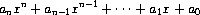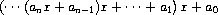# Horner's rule

Evaluating a polynomial in `x` at a given value of `x` can be formulated as an accumulation. We evaluate the polynomialusing a well-known algorithm called Horner's rule, which structures the computation asIn other words, we start with `aₙ` , multiply by `x` and so on, until we reach `a₀` . Fill in the following template to produce a procedure that evaluates a polynomial using Horner's rule. Assume that the coefficients of the polynomial are arranged in a sequence, from `a₀` through `aₙ` .

``````(define (horner-eval x coefficient-sequence)
(accumulate (lambda (this-coeff higher-terms) <??>)
0
coefficient-sequence))
``````

For example, to compute `1 + 3x + 5x³ + x⁵` at `x = 2` you would evaluate

``````(horner-eval 2 (list 1 3 0 5 0 1))
``````